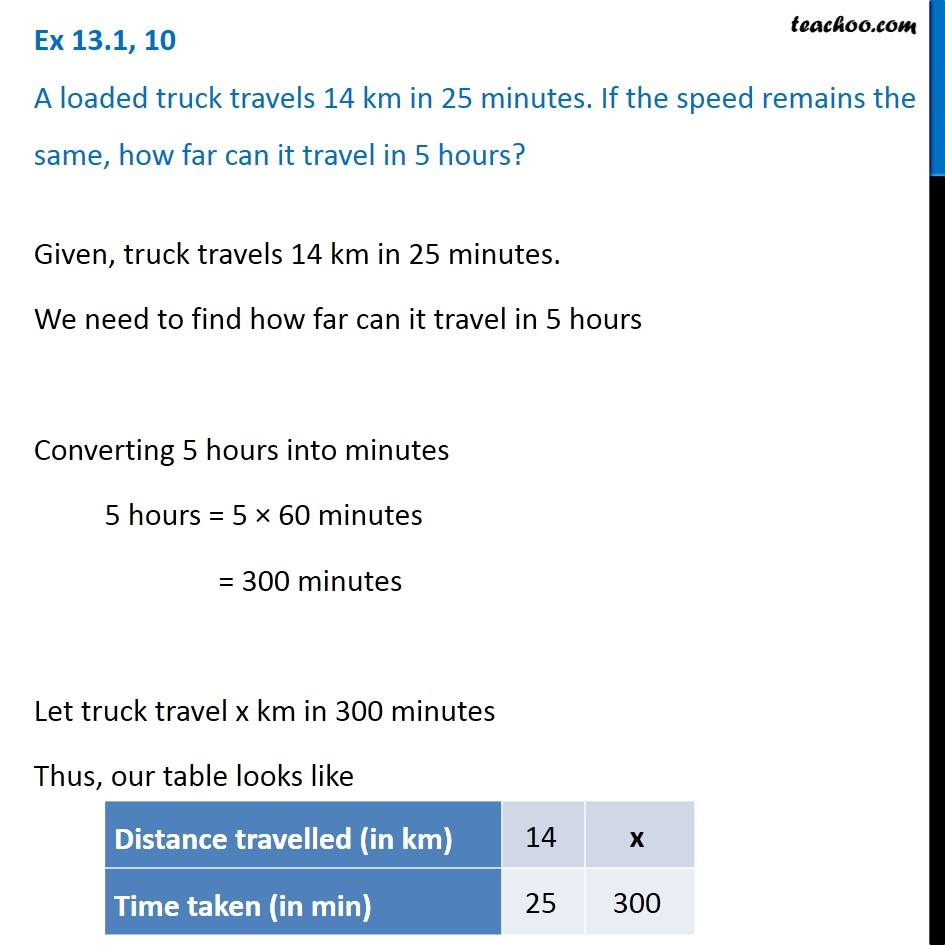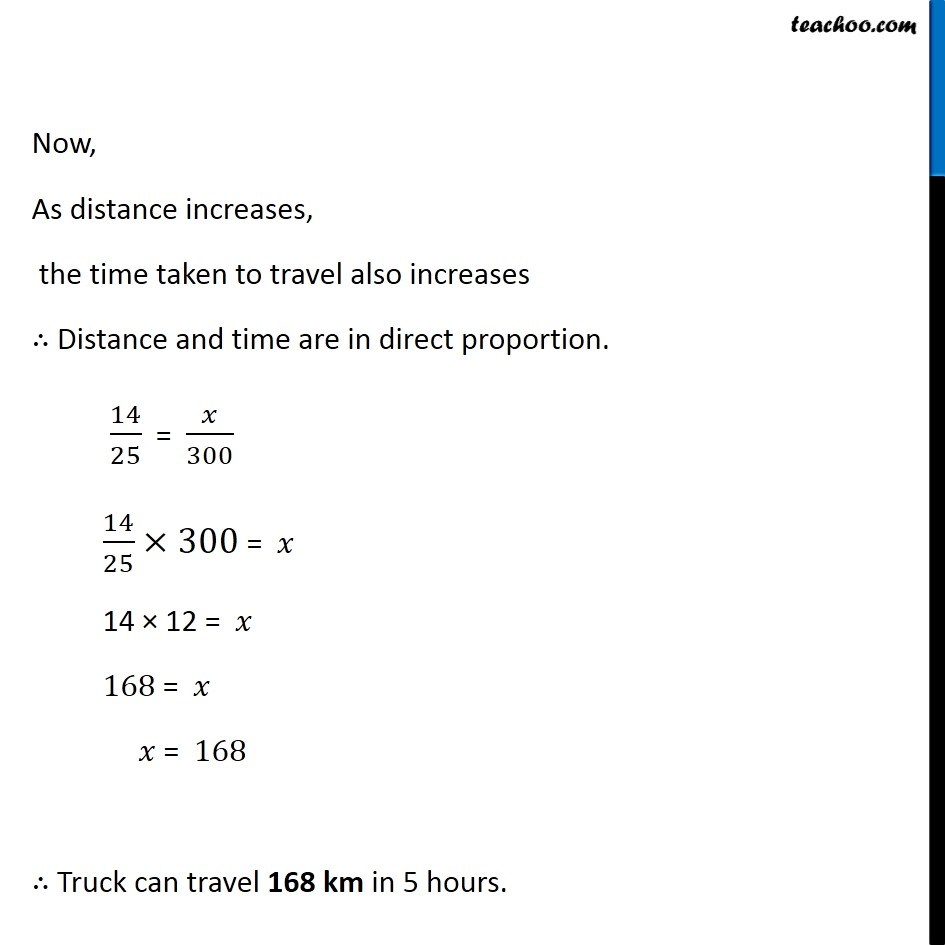1. Chapter 13 Class 8 Direct and Inverse Proportions
2. Concept wise
3. Direct Proportion

Transcript

Ex 13.1, 10 A loaded truck travels 14 km in 25 minutes. If the speed remains the same, how far can it travel in 5 hours? Given, truck travels 14 km in 25 minutes. We need to find how far can it travel in 5 hours Converting 5 hours into minutes 5 hours = 5 × 60 minutes = 300 minutes Let truck travel x km in 300 minutes Thus, our table looks like Now, As distance increases, the time taken to travel also increases ∴ Distance and time are in direct proportion. 14/25 = 𝑥/300 14/25×300 = 𝑥 14 × 12 = 𝑥 168 = 𝑥 𝑥 = 168 ∴ Truck can travel 168 km in 5 hours.

Direct Proportion